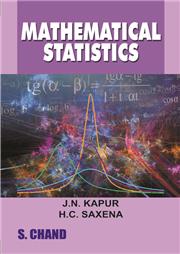Home > Higher Education > Mathematics > Mathematical Statistics# Mathematical Statistics, 2/e

## H.C. Saxena & J. N. Kapur

 ISBN : 9788121912464 Pages : 792 Binding : Perfect Language : English Imprint : S. Chand Publishing Trim size : 6.75''X9.5'' inches Weight : 1.5kg © year : 1960
 List Price :440.00
 Offer Price :352.00

20% OFF

# In Stock

Credit Card / Debit Card / Internet BankingMathematical Statistics Introduction | Frequency Distributions And Measures Of Location Measures Of Dispersions, Skewness & Kurtosis, Moments Of Frequency Distributions| Theory Of Probability | Discrete Probability Distributions | Special Discrete Probability Distributions | Univariate Continuous Probability Distributions| Special Continuous Probability Distributions | Principle Of Least Squares, Fittings Of Curves & Orthogonal Polynomials | Correlation And Regression| Multiple And Partial Correlation | Theory Of Sampling | Exact Sampling Distributions | Tests Of Significance Based On T, F And Z Distributions| Tests Of Significance Based On The Chi-Square Distribution | Statistical Theory Of Point Estimation | Testing Of Hypotheses, Sequential Analysis Distribution -Free Methods, Statistical Decision Theory | Elements Of Stochastic Processes | Order Statistics | Appendices I To Iii,| Index | Log Tables| Statistical Tables440.00352.00